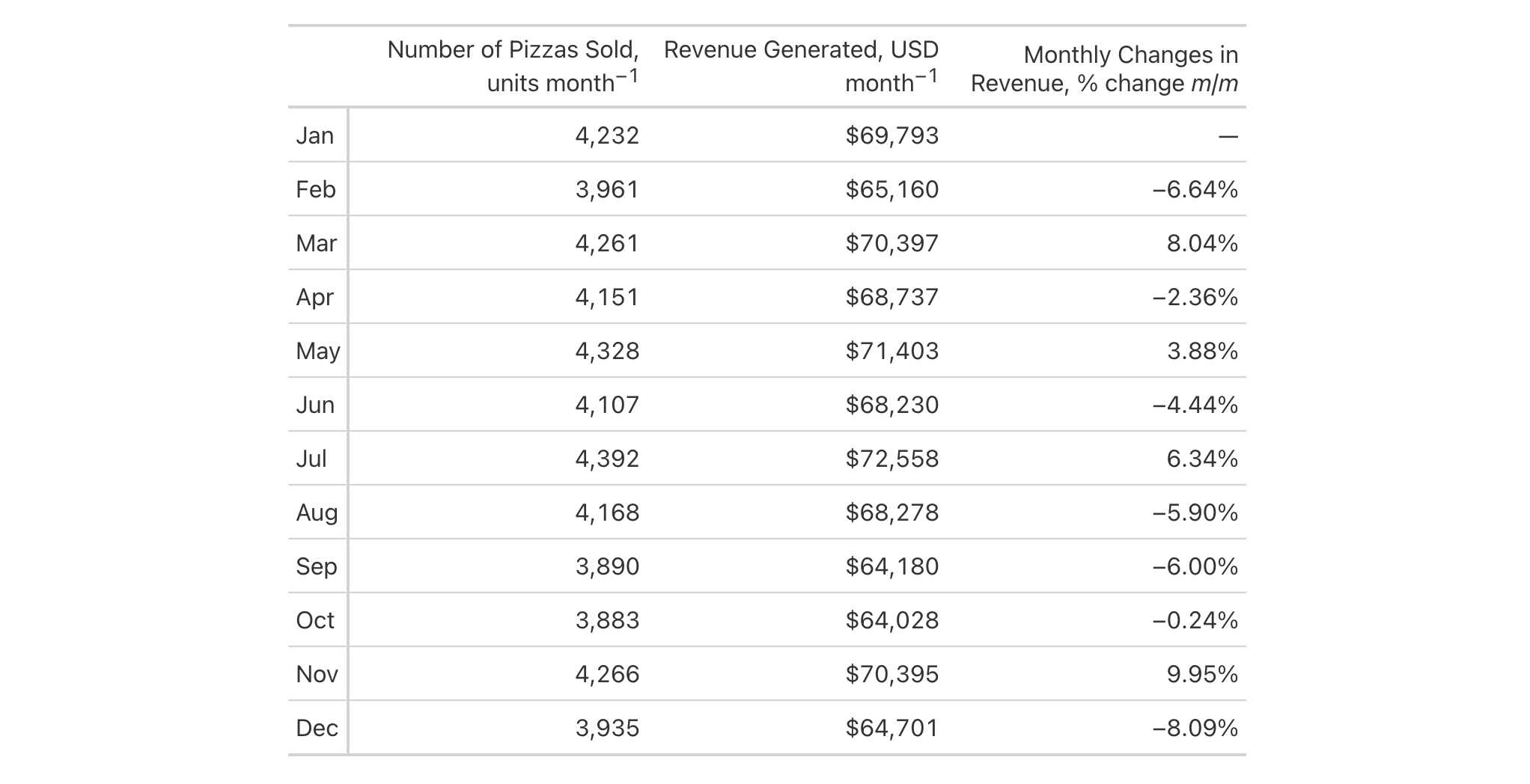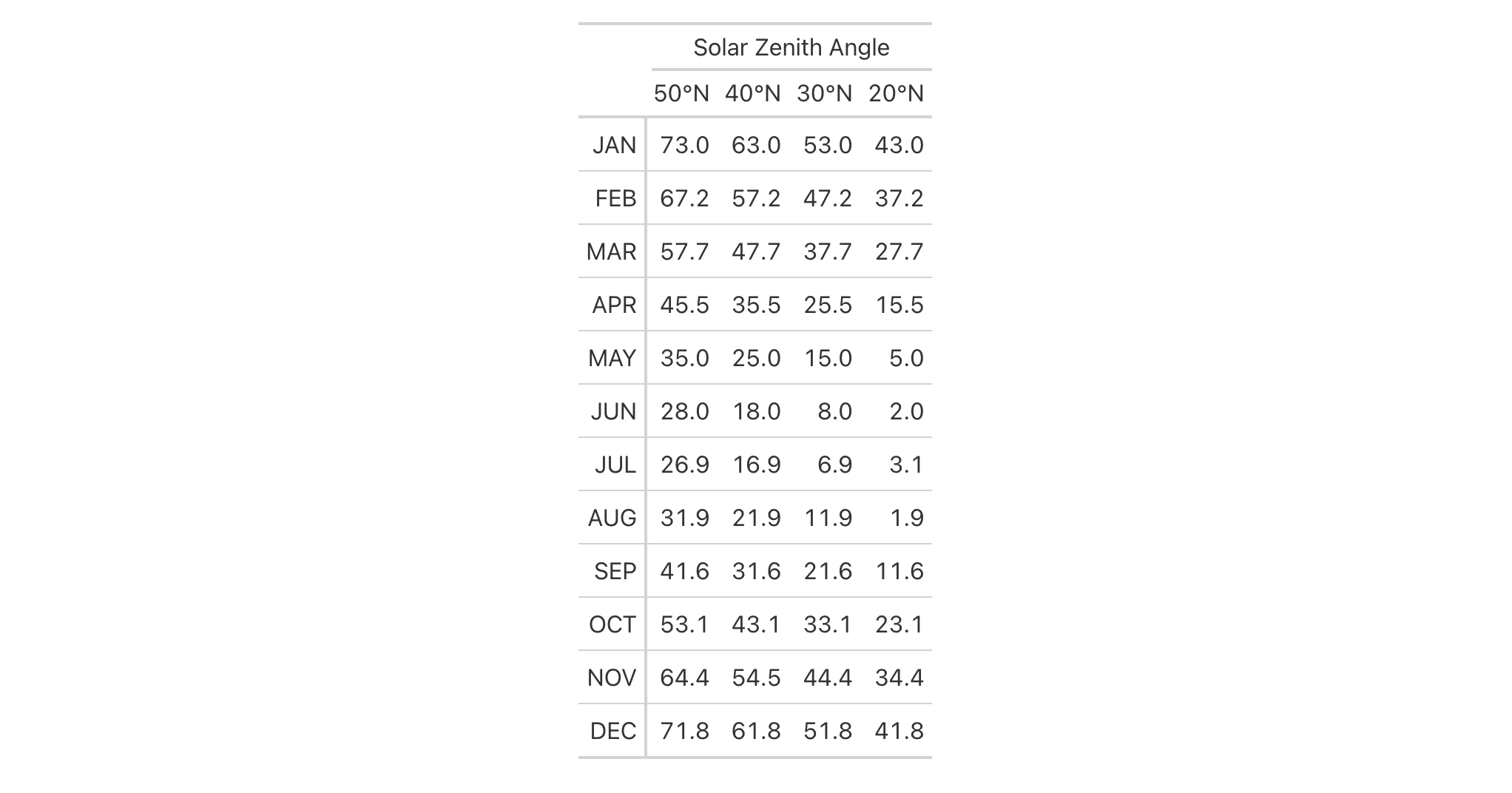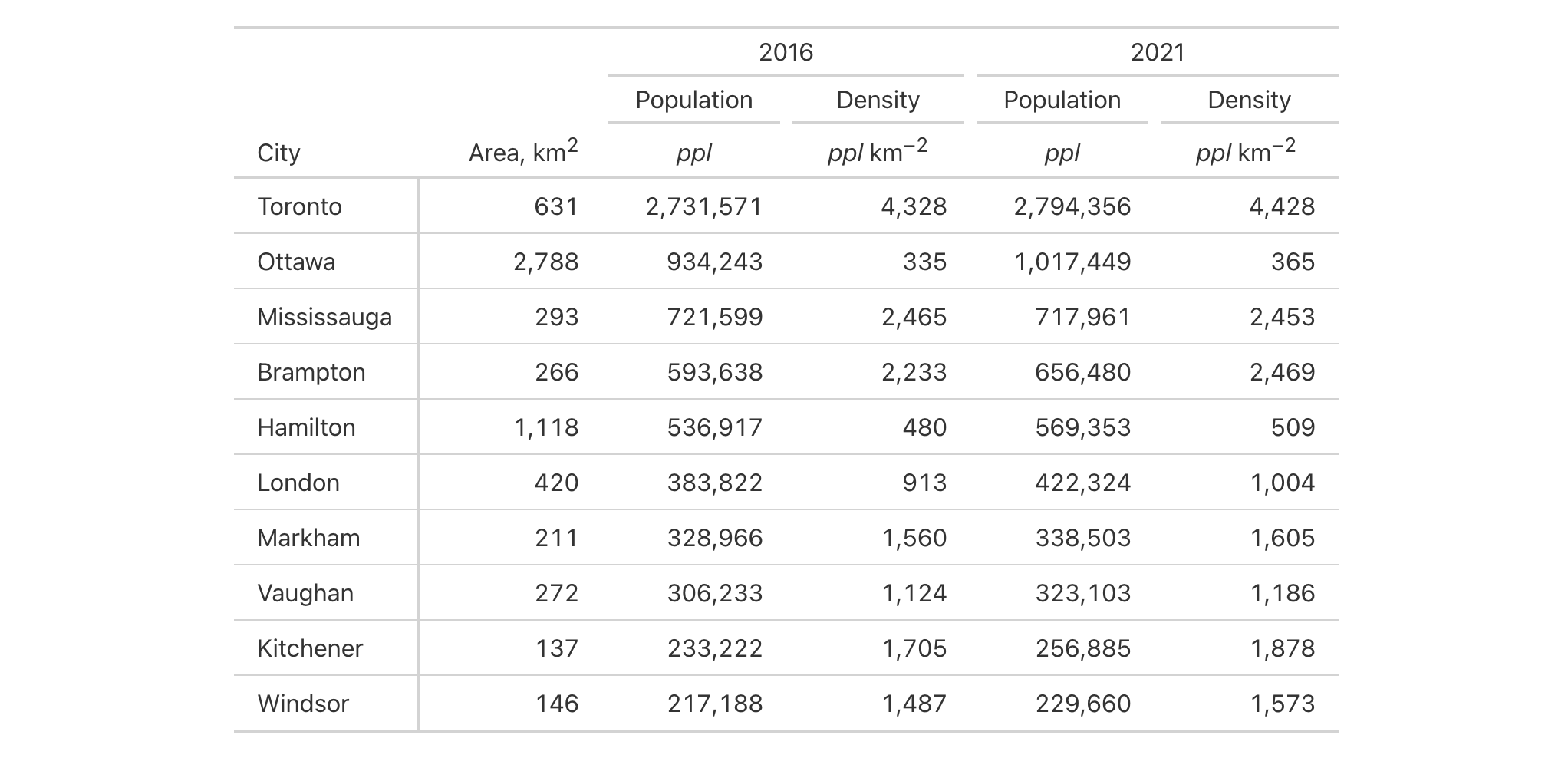Column labels can sometimes contain measurement units, and these might range from easy to define and typeset (e.g., "m/s") to very difficult. Such difficulty can arise from the need to include subscripts or superscripts, non-ASCII symbols, etc. The cols_units() function tries to make this task easier by letting you apply text pertaining to units to various columns. This takes advantage of gt's specialized units notation (e.g., "J Hz^-1 mol^-1" can be used to generate units for the molar Planck constant). The notation here provides several conveniences for defining units, letting you produce the correct formatting no matter what the table output format might be (i.e., HTML, LaTeX, RTF, etc.). Details pertaining to the units notation can be found in the section entitled How to use gt's units notation.

## Usage

cols_units(.data, ..., .list = list2(...), .units_pattern = NULL)

## Arguments

.data

The gt table data object

obj:<gt_tbl> // required

This is the gt table object that is commonly created through use of the gt() function.

...

Column units definitions

<multiple expressions> // required (or, use .list)

Expressions for the assignment of column units for the table columns in .data. Two-sided formulas (e.g., <LHS> ~ <RHS>) can be used, where the left-hand side corresponds to selections of columns and the right-hand side evaluates to single-length values for the units to apply. Column names should be enclosed in c(). Select helpers like starts_with(), ends_with(), contains(), matches(), one_of(), and everything() can be used in the LHS. Named arguments are also valid as input for simple mappings of column name to the gt units syntax; they should be of the form <column name> = <units text>. Subsequent expressions that operate on the columns assigned previously will result in overwriting column units defintion values.

.list

Alternative to ...

<list of multiple expressions> // required (or, use ...)

Allows for the use of a list as an input alternative to ....

.units_pattern

Pattern to combine column labels and units

scalar<character> // default: NULL (optional)

An optional pattern to be used for combining column labels with the defined units. The default pattern is "{1}, {2}", where "{1}" refers to the column label text and "{2}" is the text related to the associated units. This default can be modified through the column_labels.units_pattern option found in tab_options(). Setting a value here will provide an override to the column_labels.units_pattern default (only for the resolved columns in the invocation of cols_units()).

## Value

An object of class gt_tbl.

## How to use gt's units notation

The units notation involves a shorthand of writing units that feels familiar and is fine-tuned for the task at hand. Each unit is treated as a separate entity (parentheses and other symbols included) and the addition of subscript text and exponents is flexible and relatively easy to formulate. This is all best shown with examples:

• "m/s" and "m / s" both render as "m/s"

• "m s^-1" will appear with the "-1" exponent intact

• "m \s" gives the the same result, as "\<unit>" is equivalent to "<unit>^-1"

• "E_h" will render an "E" with the "h" subscript

• "t_i^2.5" provides a t with an "i" subscript and a "2.5" exponent

• "m[_0^2]" will use overstriking to set both scripts vertically

• "g/L %C6H12O6%" uses a chemical formula (enclosed in a pair of "%" characters) as a unit partial, and the formula will render correctly with subscripted numbers

• Common units that are difficult to write using ASCII text may be implicitly converted to the correct characters (e.g., the "u" in "ug", "um", "uL", and "umol" will be converted to the Greek mu symbol; "degC" and "degF" will render a degree sign before the temperature unit)

• We can transform shorthand symbol/unit names enclosed in ":" (e.g., ":angstrom:", ":ohm:", etc.) into proper symbols

• The components of a unit (unit name, subscript, and exponent) can be fully or partially italicized/emboldened by surrounding text with "*" or "**"

## Examples

Let's analyze some pizzaplace data with dplyr and then make a gt table. Here we are separately defining new column labels with cols_label() and then defining the units (to combine to those labels) through cols_units(). The default pattern for combination is "{1}, {2}" which is acceptable here.

pizzaplace |>
dplyr::mutate(month = lubridate::month(date, label = TRUE, abbr = TRUE)) |>
dplyr::group_by(month) |>
dplyr::summarize(
n_sold = dplyr::n(),
rev = sum(price)
) |>
dplyr::mutate(chg = (rev - dplyr::lag(rev)) / dplyr::lag(rev)) |>
dplyr::mutate(month = as.character(month)) |>
gt(rowname_col = "month") |>
fmt_integer(columns = n_sold) |>
fmt_currency(columns = rev, use_subunits = FALSE) |>
fmt_percent(columns = chg) |>
sub_missing() |>
cols_label(
n_sold = "Number of Pizzas Sold",
rev = "Revenue Generated",
chg = "Monthly Changes in Revenue"
) |>
cols_units(
n_sold = "units month^-1",
rev = "USD month^-1",
chg = "% change *m*/*m*"
) |>
cols_width(
stub() ~ px(40),
everything() ~ px(200)
)The sza dataset has a wealth of information and here we'll generate a smaller table that contains the average solar zenith angles at noon for different months and at different northern latitudes. The column labels are numbers representing the latitudes and it's convenient to apply units of 'degrees north' to each of them with cols_units(). The extra thing we wanted to do here was to ensure that the units are placed directly after the column labels, and we do that with .units_pattern = "{1}{2}". This append the units ("{2}") right to the column label ("{1}").

sza |>
dplyr::filter(tst == "1200") |>
dplyr::select(-tst) |>
dplyr::arrange(desc(latitude)) |>
tidyr::pivot_wider(
names_from = latitude,
values_from = sza
) |>
gt(rowname_col = "month") |>
cols_units(
everything() ~ ":degree:N",
.units_pattern = "{1}{2}"
) |>
tab_spanner(
label = "Solar Zenith Angle",
columns = everything()
) |>
text_transform(
fn = toupper,
locations = cells_stub()
) |>
tab_style(
style = cell_text(align = "right"),
locations = cells_stub()
)Taking a portion of the towny dataset, let's use spanners to describe what's in the columns and use only measurement units for the column labels. The columns labels that have to do with population and density information will be replaced with units defined in cols_units(). We'll use a .units_pattern value of "{2}", which means that only the units will be present (the "{1}", representing the column label text, is omitted). Spanners added through several invocations of tab_spanner() will declare what the last four columns contain.

towny |>
dplyr::select(
name, land_area_km2,
ends_with("2016"), ends_with("2021")
) |>
dplyr::arrange(desc(population_2021)) |>
gt(rowname_col = "name") |>
fmt_integer() |>
cols_label(
land_area_km2 ~ "Area, {{km^2}}",
starts_with("population") ~ "",
starts_with("density") ~ ""
) |>
cols_units(
starts_with("population") ~ "*ppl*",
starts_with("density") ~ "*ppl* km^-2",
.units_pattern = "{2}"
) |>
tab_spanner(
label = "Population",
columns = starts_with("population"),
gather = FALSE
) |>
tab_spanner(
label = "Density",
columns = starts_with("density"),
gather = FALSE
) |>
tab_spanner(
label = "2016",
columns = ends_with("2016"),
gather = FALSE
) |>
tab_spanner(
label = "2021",
columns = ends_with("2021"),
gather = FALSE
) |>
tab_style(
style = cell_text(align = "center"),
locations = cells_column_labels(
c(starts_with("population"), starts_with("density"))
)
) |>
cols_width(everything() ~ px(120)) |>
opt_horizontal_padding(scale = 3)5-6

## Function Introduced

In Development

Other column modification functions: cols_add(), cols_align_decimal(), cols_align(), cols_hide(), cols_label_with(), cols_label(), cols_merge_n_pct(), cols_merge_range(), cols_merge_uncert(), cols_merge(), cols_move_to_end(), cols_move_to_start(), cols_move(), cols_nanoplot(), cols_unhide(), cols_width()